• hog特征提取
千次阅读
2022-03-11 13:32:44
import math

import cv2
import numpy as np
from matplotlib import pyplot as plt

filename = './positive_sample/1.jpg'
cv2.imshow("img", img)
img1 = np.sqrt(img * 1.0 / float(np.max(img)))  # 归一化和gamma校正，取值为1.0
# cv2.imshow("img1", img1)
print(img1.shape)
cv2.namedWindow("img", 0)
cv2.waitKey(0)
# 计算梯度
height, width = img1.shape
gradient_value_x = cv2.Sobel(img1, cv2.CV_64F, 1, 0, ksize=5)  # 1代表在x方向求导
gradient_value_y = cv2.Sobel(img1, cv2.CV_64F, 0, 1, ksize=5)

# 为每个细胞单元构建梯度方向直方图， 将图像分为若干个单元格cell，默认cell为8*8的像素
cell_size = 8  # cell为8*8的细胞单元
bin_size = 8  # 直方图的条形的个数
angle_unit = 360 // bin_size
cell_gradient_vector = np.zeros((height // cell_size, width // cell_size, bin_size))

# 为每个细胞单元构建梯度方向直方图，将cell梯度与bin联系在一起，将cell写到直方图中
orientation_centers =  * bin_size  # 建立需填充矩阵
for k in range(cell_magnitude.shape):  # 遍历每个cell的高
for l in range(cell_magnitude.shape):  # 遍历每个cell的宽
min_angle = int(gradient_angle // angle_unit) % bin_size  # 找到该角度处于bin_size角度范围的最小区间
max_angle = (min_angle + 1) % bin_size  # 找到该角度处于bin_size角度范围的最大区间
orientation_centers[min_angle] += (gradient_strength * (1 - (mod // angle_unit)))
orientation_centers[max_angle] += (gradient_strength * (mod // angle_unit))
return orientation_centers

# 计算cell的大小和方向，采集细胞单元的各个像素点的梯度值和方向
# 这里两个循环，相当于给每个cell添加了bin_size维度的特征，简单来说就是求解每个cell对应角度范围的梯度值的累加
for i in range(cell_gradient_vector.shape):  # 遍历cell的高
for j in range(cell_gradient_vector.shape):  # 遍历cell的宽
cell_magnitude = gradient_magnitude[i * cell_size: (i + 1) * cell_size, j * cell_size: (j + 1) * cell_size]
cell_angle = gradient_angle[i * cell_size: (i + 1) * cell_size, j * cell_size: (j + 1) * cell_size]
# print(cell_angle.max())

# 可视化cell的梯度直方图
hog_image = np.zeros([height, width])
cell_width = cell_size // 2
angle = 0
angle_gap = angle_unit
x1 = int(x * cell_size + magnitude * cell_width * math.cos(angle_radian))
y1 = int(y * cell_size + magnitude * cell_width * math.sin(angle_radian))
x2 = int(x * cell_size - magnitude * cell_width * math.cos(angle_radian))
y2 = int(y * cell_size - magnitude * cell_width * math.sin(angle_radian))
cv2.line(hog_image, (y1, x1), (y2, x2), int(255 * math.sqrt(magnitude)))
angle += angle_gap
plt.imshow(hog_image, cmap=plt.cm.gray)
plt.show()

# 统计block梯度信息，把cell单元组合成更大的块，块内归一化梯度直方图
hog_vector = []
for i in range(cell_gradient_vector.shape - 1):
for j in range(cell_gradient_vector.shape - 1):
block_vector = []
mag = lambda vector: math.sqrt(sum(i ** 2 for i in vector))
magnitue = mag(block_vector)
if magnitude != 0:
normalize = lambda block_vector, magnitude:[element // magnitude for element in block_vector]
block_vector = normalize(block_vector, magnitude)
hog_vector.append(block_vector)
print(np.array(hog_vector).shape)  # (4661,32),共有4661个block，每个black都有32维的特征

cv2.waitKey(0)


import math
import os
from itertools import chain

import cv2
import joblib
import numpy as np
import skimage.novice
from PIL import Image
from matplotlib import pyplot as plt
from sklearn.svm import LinearSVC

class Hog_feature_extraction():
def __init__(self, img, cell_size=8, bin_size=8):
self.img = img
self.img = np.sqrt(img / float(np.max(img)))
self.cell_size = cell_size
self.bin_size = bin_size
self.angle_unit = 360 // bin_size
assert type(self.bin_size) == int, "bin_size should be integer,"
assert type(self.cell_size) == int, "cell_size should be integer,"
assert type(self.angle_unit) == int, "bin_size should be divisible by 360"

# 1-计算cell梯度；3-可视化直方图；
def caculate_block(self, img):
gradient_value_x = cv2.Sobel(img, cv2.CV_64F, 1, 0, ksize=5)  # 1代表在x方向求导
gradient_value_y = cv2.Sobel(img, cv2.CV_64F, 0, 1, ksize=5)
cell_gradient_vector = np.zeros((height // self.cell_size, width // self.cell_size, self.bin_size))
for i in range(cell_gradient_vector.shape):  # 遍历cell的高
for j in range(cell_gradient_vector.shape):  # 遍历cell的宽
cell_magnitude = gradient_magnitude[i * self.cell_size: (i + 1) * self.cell_size, j * self.cell_size: (j + 1) * self.cell_size]
cell_angle = gradient_angle[i * self.cell_size: (i + 1) * self.cell_size, j * self.cell_size: (j + 1) * self.cell_size]
# 可视化直方图
cell_width = self.cell_size // 2
hog_image = np.zeros([height, width])
angle = 0
angle_gap = self.angle_unit
x1 = int(x * self.cell_size + magnitude * cell_width * math.cos(angle_radian))
y1 = int(y * self.cell_size + magnitude * cell_width * math.sin(angle_radian))
x2 = int(x * self.cell_size - magnitude * cell_width * math.cos(angle_radian))
y2 = int(y * self.cell_size - magnitude * cell_width * math.sin(angle_radian))
cv2.line(hog_image, (y1, x1), (y2, x2), int(255 * math.sqrt(magnitude)))
angle += angle_gap
hog_vector = []
for i in range(cell_gradient_vector.shape - 1):
for j in range(cell_gradient_vector.shape - 1):
block_vector = []
mag = lambda vector: math.sqrt(sum(i ** 2 for i in vector))
magnitue = mag(block_vector)
if magnitude != 0:
normalize = lambda block_vector, magnitude: [element // magnitude for element in block_vector]
block_vector = normalize(block_vector, magnitude)  # block混叠空间块归一化
hog_vector.append(block_vector)
return hog_image, hog_vector  # 特征描述子

# 2-构建直方图；
def cell_gradient(self, cell_magnitude, cell_angle):  # 将同一个cell的梯度值根据分的角度值用一权重分别赋给bin_size个维度
orientation_centers =  * self.bin_size  # 建立需填充矩阵
for k in range(cell_magnitude.shape):  # 遍历每个cell的高
for l in range(cell_magnitude.shape):  # 遍历每个cell的宽
min_angle = int(gradient_angle // self.angle_unit) % self.bin_size  # 找到该角度处于bin_size角度范围的最小区间
max_angle = (min_angle + 1) % self.bin_size  # 找到该角度处于bin_size角度范围的最大区间
orientation_centers[min_angle] += (gradient_strength * (1 - (mod // self.angle_unit)))
orientation_centers[max_angle] += (gradient_strength * (mod // self.angle_unit))
return orientation_centers

height, width = img.shape
pos_hog = Hog_feature_extraction(img, cell_size=8, bin_size=8)
hog_image, pos_hog_vector = pos_hog.caculate_block(img)  # 获取图像的hog特征，blockpython 计算机视觉 机器学习
更多相关内容
• 基于python的HOG特征提取算法代码设计与实现
• hog特征提取matlab代码字符图像识别MATLAB 图像预处理（二值化），定向梯度直方图（HOG特征提取，SVM调整VA网格搜索（内核，内核比例，BoxConstraint），分类分析（混淆矩阵）。 在Matlab中运行代码之前，请加载...
• 基于HOG特征提取的图像分类器，HOG的核心思想是所检测的局部物体外形能够被光强梯度或边缘方向的分布所描述。通过将整幅图像分割成小的连接区域称为cells，每个cell生成一个方向梯度直方图或者cell中pixel的边缘方向...
• CudaHoG Dalal & Triggs Histogram of Oriented Gradients  特征提取器在 CUDA 中的高效实现。 该库是可扩展的，使用 CImg 并用 C++11 编写。 该库还通过 MEX 函数（仅限 Linux 版本）提供 MATLAB 绑定。编译使用...C++
• 基于opencv的HOG特征提取，代码使用vs2010与opencv实现，可提取人脸的HOG特征，代码可直接运行。
• 文件里有matlab编写的HOG特征提取代码，还有Lena图和我的实验结果图
• HOG特征提取，以及特征编码，相关HOG特征的讲解。。。。
• hog特征提取matlab代码BacteriaImageProcess 这是为了我们的项目：分割细菌图像并识别该图像中的细菌种类。 从本质上讲，这是对细菌数据的深度学习应用程序。 我们在matlab中实现了卷积RBM，以完成我们的任务。 cdbn...
• HOG特征提取过程为: Gamma归一化； 计算梯度； 划分cell 组合成block，统计block直方图； 梯度直方图归一化； 收集HOG特征。 Gamma归一化: 对图像颜色进行Gamma归一化处理，降低局部阴影及背景因素的影响． 计算...
• hog特征提取matlab代码用于图像分类的计算机视觉特征提取工具箱 该工具箱的目的是简化用于图像分类相关任务的常用计算机视觉功能（例如HOG，SIFT，GIST和Color）的特征提取过程。 包含的功能的详细信息在中提供。 ...
• 图像HOG特征提取算法MATLAB仿真，matlab2021a运行测试
• hog特征提取，从原理上分析，最后生成一副特征
• % HOG特征提取------------ % 第1步：计算水平,竖直方向, 像素梯度矩阵：Ix、Ty % 第2步：计算image对应：angle,magnitude矩阵 % 第3步：遍历, 第1层遍历block, 第2层遍历cell, 第3层遍历pixel % 计算每个pixel...
• hog特征提取，空间描述 anna_PHOG Computes Pyramid Histogram of Oriented Gradient over a ROI. % % [BH, BV] = anna_PHOG(I,BIN,ANGLE,L,ROI) computes phog descriptor over a ROI. % % Given and image I, ...
• 提取输入图像的HOG特征，输出灰度图、校正图和处理好的梯度图，得到HOG特征做下一步的处理
• 方向梯度直方图检测算子，能够提取图像的HOG特征，进行分类与识别
• 可运行的HOG特征提取，参数已设定，可更改，清晰易懂
• 方向梯度直方图（Histogram of Oriented Gradient, HOG特征是一种在计算机视觉和图像处理中用来进行物体检测的特征描述子。它通过计算和统计图像局部区域的梯度方向直方图来构成特征Hog特征结合SVM分类器已经被...

# 原理

其实本质就是梯度的统计信息，而梯度主要存在于边缘的地方。在一副图像中，局部目标的表象和形状（appearance and shape）能够被梯度或边缘的方向密度分布很好地描述。

# 方法

Hog特征提取主要依靠于三个参数： 检测窗口、块（block）、细胞单元。假定提取参数为      winSize(64,128), blockSize(16,16), blockStride(8,8), cellSize(8,8)，nbins(9)
其中nBins表示在一个胞元（cell）中统计梯度的方向数目，例如nBins=9时，在一个胞元内统计9个方向的梯度直方图，每个方向为180/9=20度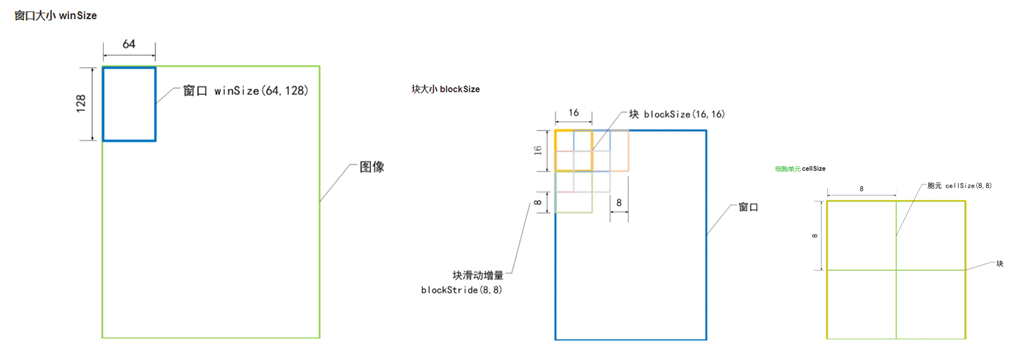Hog特征提取中最小的统计单位，我们把它叫细胞单元（cell）。采集细胞单元中各像素点的梯度的或边缘的方向直方图。最后把这些直方图组合起来就可以构成特征描述器。

性能提升 ：把每个Block进行对比度归一化（contrast-normalized），所采用的方法是：先计算各直方图在这个区间（block）中的密度，然后根据这个密度对区间中的各个细胞单元做归一化。通过这个归一化后，能对光照变化和阴影获得更好的效果。具体步骤中第5点所述。

# 具体步骤

HOG提取流程图如下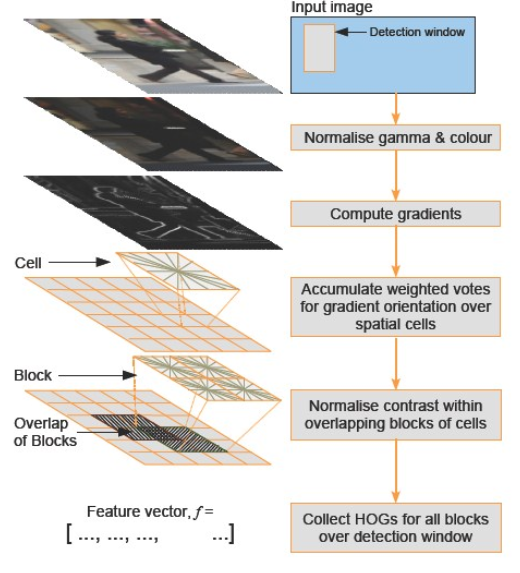以一个检测窗口提取HOG为例
1. 灰度化
2.Gamma归一化

采用Gamma校正法对输入图像进行颜色空间的标准化（归一化）；目的是调节图像的对比度，降低图像局部的阴影和光照变化所造成的影响，同时可以抑制噪音的干扰；
其实就是对像素点做 幂率 处理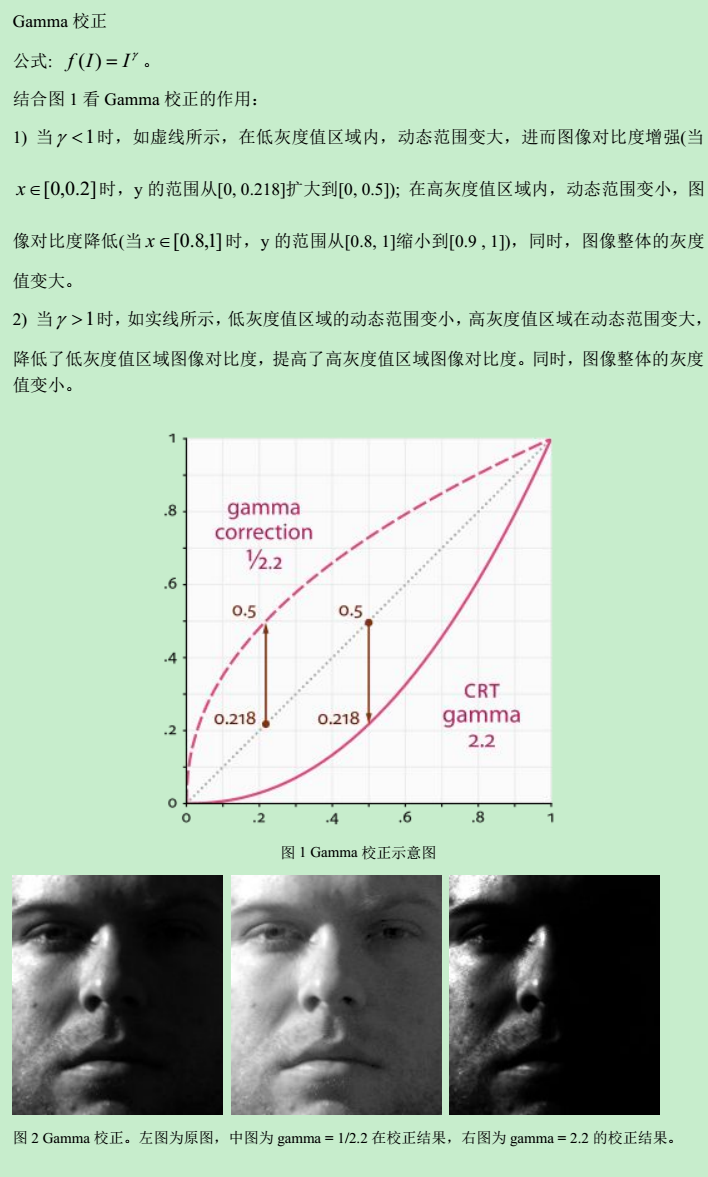假设图像中有一个像素，值是 200 ，那么对这个像素进行校正必须执行如下步骤：
1. 归一化 ：将像素值转换为  0 ～ 1  之间的实数。 算法如下 : ( i + 0. 5)/256  这里包含 1 个除法和 1 个加法操作。对于像素  A  而言  , 其对应的归一化值为  0. 783203 。
2. 预补偿 ：根据公式  , 求出像素归一化后的 数据以  1 /gamma  为指数的对应值。这一步包含一个 求指数运算。若  gamma  值为  2. 2 ,  则  1 /gamma  为  0. 454545 , 对归一化后的  A  值进行预补偿的结果就 是  0. 783203 ^0. 454545 = 0. 894872 。
3. 反归一化 ：将经过预补偿的实数值反变换为  0  ～  255  之间的整数值。具体算法为 : f*256 - 0. 5  此步骤包含一个乘法和一个减法运算。续前 例  , 将  A  的预补偿结果  0. 894872  代入上式  , 得到  A  预补偿后对应的像素值为  228 , 这个  228  就是最后送 入显示器的数据。

3.计算梯度

计算图像横坐标和纵坐标方向的梯度，并据此计算每个像素位置的梯度方向值；求导操作不仅能够捕获轮廓，人影和一些纹理信息，还能进一步弱化光照的影响 。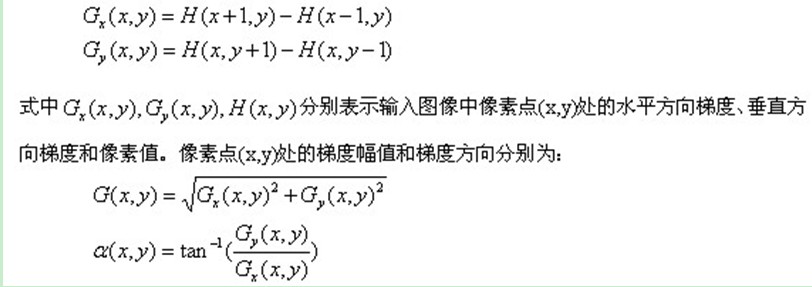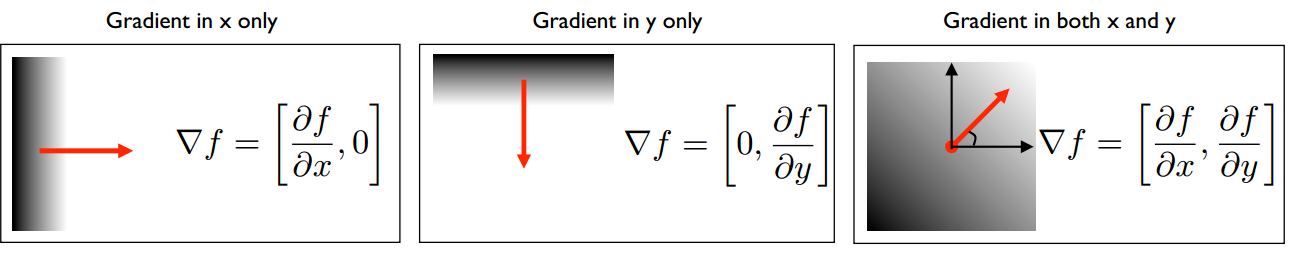4. 构建细胞单元（Cell）的梯度方向直方图

例如上面所说的假定一个细胞单元是8*8像素，则整个单元内共有64个像素点的梯度信息，我们需要对这个Cell做梯度直方图统计。如前所述例如nBins=9时，在一个胞元内统计9个方向的梯度直方图。因为如上公式tan的函数周期为兀,所以直方图表述的范围在0-180度间，180/9=20度，如下图示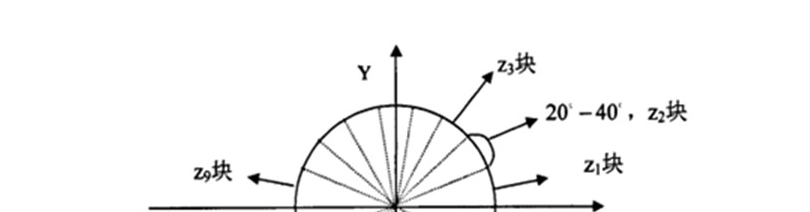梯度大小就是作为投影的权值，比如某个像素的梯度方向是20-40度，然后它的梯度大小是100， 那么直方图第2个bin的计数就在原基础上+100.

5.Block梯度强度归一化
之前就提到了，多个cell组成一个block。
由于局部光照的变化以及前景-背景对比度的变化，使得梯度强度的变化范围非常大。这就需要对梯度强度做归一化。归一化能够进一步地对光照、阴影和边缘进行压缩。
把各个细胞单元组合成大的、空间上连通的区间（blocks）。这样，一个block内所有cell的特征向量串联起来便得到该block的HOG特征。这些区间是互有重叠的，这就意味着：每一个单元格的特征会以不同的结果多次出现在最后的特征向量中。我们将归一化之后的块描述符（向量）就称之为HOG描述符。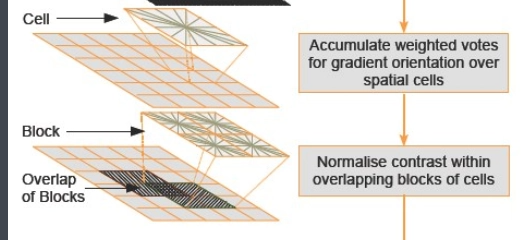6.收集HOG特征

最后一步就是将检测窗口中所有重叠的块进行HOG特征的收集，并将它们结合成最终的特征向量供分类使用。

7.一个图像的HOG特征维数总共有多少呢？

对于128*64的图像，cell为8*8，2*2个cell组成一个block,则block为16*16，每个像素9个梯度方向，则每个block内有 9*2*2=36，滑动步长为8像素，则水平方向有128/8 -1 =  15个扫描窗口，垂直方向有64/8 -1 = 7个扫描窗口，所以 总共有 36 * 15 * 7 = 3780个特征
所以HOG特征维数 取决于一个block内有多少个cell和滑动步长

展开全文梯度直方图 SVM 机器学习
• HOG检测器默认属性，RGB颜色空间，无伽马校正； [−1,0,1]梯度滤波器，无平滑；线性梯度投票在0◦–180◦中的9个方向仓中；四个8×8像素单元的16×16像素块; σ= 8像素的高斯空间窗； L2-Hys（Lowe样式修剪的L2范数...

# HOG概述

HOG (Histogram of Oriented Gradients),即方向梯度的直方图.它统计某个方向区间内的梯度大小(即 voting vector,投票矢量).核心思想是把每个图像模块划分为固定大小的8X8单元格, 描述梯度的幅度和方向.将每个模块对应的HOG特征描述为重要的跟踪信息，再通过Gamma去高光等处理准确提取的图像的特征信息。
即在所有的64个单元格上单独计算直方图,指定x轴设置的区间数, x轴表示梯度方向,它的范围指定为0~180°.图1 梯度方向分割
计算直方图的函数代码实现

'''
函数名称：calc_hist
功能：计算直方图
输入：
mag    幅值矩阵
angle  角度矩阵，范围在 0-180
bin_size    直方图区间大小
输出：
hist    直方图
'''
def calc_hist(mag, angle, bin_size=9):
hist = np.zeros((bin_size,), dtype=np.int32)

bin_step = 180 // bin_size
bins = (angle // bin_step).flatten()
flat_mag = mag.flatten()

for i,m in zip(bins, flat_mag):
hist[i] += m

return hist


之后计算单元格的部分:

# 将图像切成多个cell
cell_size = 8
bin_size = 9
img_h, img_w = gray.shape[:2]
cell_h, cell_w = (img_h // cell_size, img_w // cell_size)

cells = np.zeros((cell_h, cell_w, bin_size), dtype=np.int32)
for i in range(cell_h):
cell_row = cell_size * i
for j in range(cell_w):
cell_col = cell_size * j
cells[i,j] = calc_hist(mag[cell_row:cell_row+cell_size, cell_col:cell_col+cell_size],
angle[cell_row:cell_row+cell_size, cell_col:cell_col+cell_size], bin_size)


多个单元格组合成一个块.即图中黄色格子.每个单元格上面有一个9维的表示直方图大小的向量,那么一个块就有2X2X9=36维向量, 块就是要把每次选中的这36维向量做规范化,得到新的36维向量.规范化的方法有:通常使用L2-Norm,先对整个整个向量的各个元素都求平方然后求和、开根号 作为规范化因子，然后对原向量中每一个元素都除以这个规范化因子。
L2 规范化的函数实现代码:

# 归一化cells
def l2_norm(cells):
block = cells.flatten().astype(np.float32)
norm_factor = np.sqrt(np.sum(block**2) + 1e-6)
block /= norm_factor
return block


再利用之前得到的单元格和规范化函数就可以写块实现的操作了.

# 多个cell融合成block
block_size = 2
block_h, block_w = (cell_h-block_size+1, cell_w-block_size+1)
blocks = np.zeros((block_h, block_w, block_size*block_size*bin_size), dtype=np.float32)
for i in range(block_h):
for j in range(block_w):
blocks[i,j] = l2_norm(cells[i:i+block_size, j:j+block_size])


把这么多个 block 的 36维向量拼起来就是 HOG 特征描述子（descriptor）了，在这里来说就是把 blocks 这个 3 维的矩阵摊平，也只要一行代码：

blocks = blocks.flatten()


我把整个 HOG 的计算过程封成了一个函数，是这样的：

# 计算HOG特征
def calc_hog(gray):
''' 计算梯度 '''
dx = cv2.Sobel(gray, cv2.CV_16S, 1, 0)
dy = cv2.Sobel(gray, cv2.CV_16S, 0, 1)
sigma = 1e-3
# 计算角度
angle = np.int32(np.arctan(dy / (dx + sigma)) * 180 / np.pi) + 90
dx = cv2.convertScaleAbs(dx)
dy = cv2.convertScaleAbs(dy)
# 计算梯度大小
mag = cv2.addWeighted(dx, 0.5, dy, 0.5, 0)

print('angle\n', angle[:8,:8])
print('mag\n', mag[:8,:8])
''' end of 计算梯度 '''

# 将图像切成多个cell
cell_size = 8
bin_size = 9
img_h, img_w = gray.shape[:2]
cell_h, cell_w = (img_h // cell_size, img_w // cell_size)

cells = np.zeros((cell_h, cell_w, bin_size), dtype=np.int32)
for i in range(cell_h):
cell_row = cell_size * i
for j in range(cell_w):
cell_col = cell_size * j
cells[i,j] = calc_hist(mag[cell_row:cell_row+cell_size, cell_col:cell_col+cell_size],
angle[cell_row:cell_row+cell_size, cell_col:cell_col+cell_size], bin_size)

# 多个cell融合成block
block_size = 2
block_h, block_w = (cell_h-block_size+1, cell_w-block_size+1)
blocks = np.zeros((block_h, block_w, block_size*block_size*bin_size), dtype=np.float32)
for i in range(block_h):
for j in range(block_w):
blocks[i,j] = l2_norm(cells[i:i+block_size, j:j+block_size])

return blocks.flatten()


假设输入的图片是 64 x 128 的，cell 就会有 8 x 16 = 128个，block 就有 (8-2+1) x (16 - 2 + 1) = 105 个，每个 block 有 36 维向量，总共就是 105 x 36 = 3780维向量，这个向量就是对应这张图片的 HOG 特征。用其他特征得到的东西也是大同小异，都是不同大小表示不同信息的特征。

特征相当于该物体的 ID，如果同类的物体的特征很相似，我们就说这个特征至少对于该类物体的区分度很好。拿深度神经网络来说，用它做人脸识别的时候，也是输入图片，输出这么一个长长的向量，如果对于同一个人，这些产生的向量的距离很近，而对于不同人的距离则很远，就说这个神经网络精度很高，但本质的流程和这些人工设计的特征没有任何区别。
HOG检测器默认属性，RGB颜色空间，无伽马校正； [−1,0,1]梯度滤波器，无平滑；线性梯度投票在0◦–180◦中的9个方向仓中；四个8×8像素单元的16×16像素块; σ= 8像素的高斯空间窗； L2-Hys（Lowe样式修剪的L2范数）块归一化；块间​​距为8个像素（因此每个单元的覆盖率为4倍）； 64×128检测窗口；线性SVM分类器。
图1总结了各种HOG参数对整体检测性能的影响。这些将在下面详细讨论。主要结论是，为了获得良好的性能，应使用精细比例的导数（基本上不进行平滑处理），多个方向框以及中等大小的，高度归一化的重叠描述符块。图1 .中 （a）使用精细的导数比例可以显着提高性能。 （“ c-cor”是一维三次校正点导数）。 （b）增加定向箱的数量可显着提高性能，直到在0°至180°范围内间隔约9个箱。 （c）不同块归一化方案的影响。 （d）使用重叠的描述符块可将丢失率降低约5％。 （e）减少64×128检测窗口周围的16个像素边距会使性能降低约4％。 （f）使用高斯内核SVM exp（-γ？x1-x2？2）将性能提高约3％。

# 应用示例

特征区分度:
首先要介绍一下我使用的公开数据集 INRIA Person，这是一个公开的行人数据集，里面分为正样本和负样本，正样本几乎都是直立的老外行人，负样本是一些风景图片，可以给大家看一眼，这个数据集也能从网上直接下载。正样本.png负样本.png
我会把所有图片缩放到高度 128 和宽度 64，因此每张图片的 HOG 特征长度是 3780，如果我把所有这些 3780 维的向量都放在 3780 维空间上去看它们的分布，可能正样本会聚集在一堆，负样本聚在另一堆，这样是最好的，但是我们没办法可视化 3780 维的空间，所以我的做法是用 PCA（主成分分析）把它们压到二维，在二维平面上去看。
核心代码是这样的，需要 sklearn 和 scipy，可以通过 pip 安装：

from sklearn.decomposition import PCA
import matplotlib.pyplot as plt

# PCA 降维
pca = PCA(n_components=2, copy=True)
data_size = 500
pos_features = pca.fit_transform(pos_features[:data_size])
neg_features = pca.fit_transform(neg_features[:data_size])
# 显示
plt.plot(pos_features[:,0], pos_features[:,1], 'ro')
plt.plot(neg_features[:,0], neg_features[:,1], 'bo')
plt.show()


得到的图形是这样的:蓝色点是行人，红色点是背景。
emmmm, 好像打脸了，（逃
打脸的原因可能有两个，一个是降维降太多了，二维信息不足以表达原来的 3000 多维的结构；二是我们看这个图形的角度不对 ，正所谓横看成岭侧成峰。假设这是两坨饼，红色一坨蓝色一坨，现在看起来是红色的饼叠在了蓝色的饼上面，所以正确的看法应该是，我们把红色的饼拿起来，然后从侧面去看，就会变成这样：即完成了分类,线性可分.
SVM模型

from sklearn import svm

# 合并特征
features = np.concatenate((pos_features[:data_size], neg_features[:data_size]))
labels = np.zeros((data_size*2,), dtype=np.int32)
labels[:data_size] = 1

# SVM分类器
lin_clf = svm.LinearSVC()
lin_clf.fit(features, labels)


features 是正样本和负样本的特征合并起来的一个大矩阵，labels 表示的是每个特征对应的是什么类别，这里我设置了 1 对应行人，0 对应背景。为什么需要 labels，因为训练模型要用，训练模型跟老师教学生学习很像，我们要先给学生一吨的题，并且告诉他们背后有答案，自己对，这些题就是 features，答案就是 labels，于是他们做完对完这些题以后我们就希望他们能够举一反三，看到新的题的时候不方。lin_clf 就是 SVM模型，使用 fit 方法训练，稍等几秒就训练完了。
测试代码:

miao = cv2.imread('miao2.jpg')
miao = cv2.resize(miao, (64,128))
miao = cv2.cvtColor(miao, cv2.COLOR_BGR2GRAY)
miao_feature = calc_hog(miao)
pred_result = lin_clf.predict(np.array([miao_feature]))


结果 pred_result 当然是 1 了，如果不是我就不会放上来了。

参考链接::
https://www.jianshu.com/p/ed21c357ec12

展开全文python 行人识别
• HOG特征提取程序中带有详细的程序说明，方便大家理解程序...